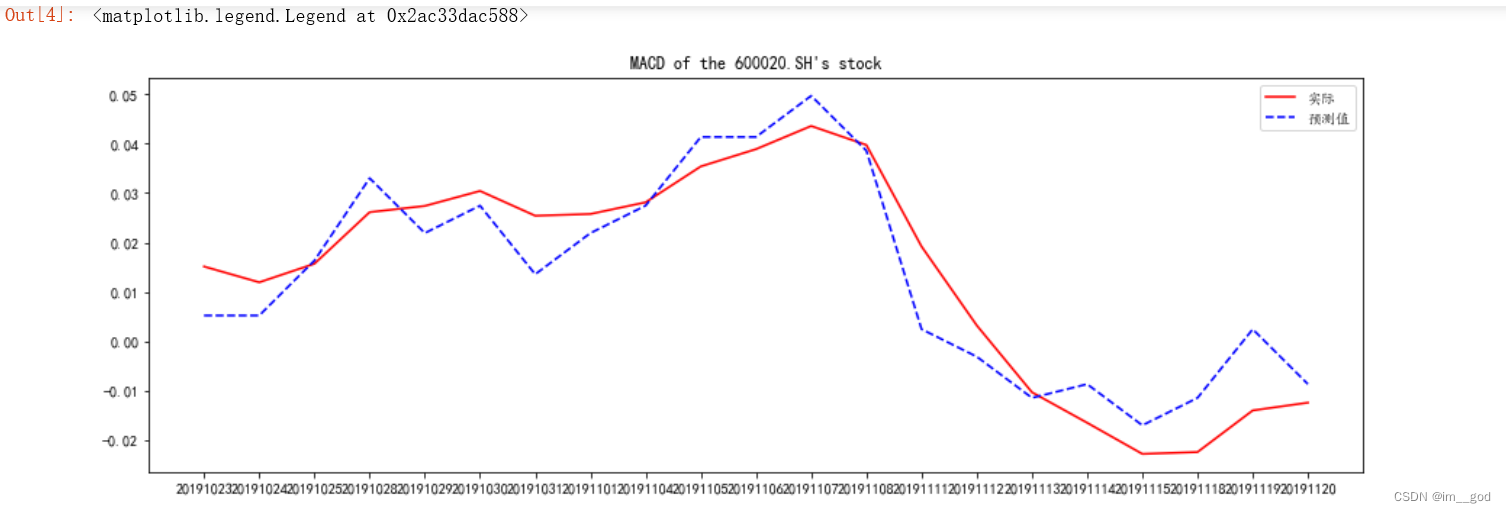• 股票预测python
千次阅读
2021-02-03 20:02:49

SVM 支持向量机

原理就不赘述了，其余的文章有讲过。SVM是一种十分优秀的分类算法，使用SVM也能给股票进行一定程度上的预测。

核心

因为是分类算法，因此不像ARIMA一样预测的是时序。分类就要有东西可分，因此将当日涨记为1，跌记为0，作为分类的依据。使用历史数据作为训练数据。

处理数据：

1.股票历史数据来源于yahoo_finance api，获取其中Open，Close，Low，High，Volume作为基础。因为除去Volume以外，其余数据都是Price，基于Price并不能很好的表达股票的特性，或者说并不太适用于SVM分类算法的特性。基于SVM算法的特性，股票并不是到达一个价格范围就有大概率涨或跌(不知道我这个表达大家能不能看懂)。

2.基于上述原因，我决定将Price转换成另一种形式的数据。例如：High-Low=全天最大价格差，Open-YesterdayOpen=当天Open价格变动，Open-YesterdayClose=开盘价格变动。(我也并不太懂经济学，仅仅是为了寻找另一种更好的方案)

3.单纯地基于历史数据是完全不够的，因此还使用了R语言和tm.plugin.sentiment包，进行语义分析，进行新闻正面负面的判定。这块不是我做的，了解的并不多。新闻并不是每天都有的，这样的话新闻数据就显得有些鸡肋，无法在分类算法中起到作用，但是我们能在多个站点中提取，或是直接将关键字定为Debt(判断大众反应)。

4.这里仅仅是进行了两个站点的新闻挖掘，然后可通过rpy2包在Python中运行R语言，或是R语言得到的数据导出成Json，Python再读取。至此，数据处理告一段落。

SVM算法：

股票数据不能完全基于历史数据，因此需要一定数量的历史数据推出预测数据，例如这边使用了70天的数据训练，来推出后一天的股票涨跌，而不是所有的历史数据。最后的成绩是53.74%的正确率，对于一个基本使用历史数据来预测股市的方法而言已经是个不错的结局了。

Github

https://github.com/jerry81333/StockProdiction/

更多相关内容
• 简单的股市预测模型，测试环境windows 10 ,anaconda4.4 ,py3.6python
• 股市预测python代码<循环神经网络> 数据集：（百度网盘） 链接：https://pan.baidu.com/s/1ozBoPhO7LU06z9mNlufbmg 提取码：59vk #基于。csv数据没建立rnn模型，预测 # 1.数据预处理，将序列数据转化为可用于...

## 股市预测python代码<循环神经网络>

数据集：（百度网盘）
链接：https://pan.baidu.com/s/1ozBoPhO7LU06z9mNlufbmg
提取码：59vk

#基于。csv数据没建立rnn模型，预测
# 1.数据预处理，将序列数据转化为可用于rnn输入的数据
# 2.对新数据进行预测（预测数据集），可视化结果
# 3.存储预测结果（csv文件），并观察局部预测结果（局限性）
#模型结构：单纯rnn，输出有五个神经元，每次使用前8个数据预测第9个数据

import pandas as pd
import numpy as np

price = data.loc[:,'close']

#归一化处理
price_norm = price/max(price)
print(price_norm)

#可视化
from matplotlib import pyplot as plt
fig1=plt.figure(figsize=(8,5))#建立图形
plt.plot(price)#画图
#加标注
plt.title('close price')
plt.xlabel('time')
plt.ylabel('price')
plt.show()

#xy的赋值

#define X and y
#define method to extract X and y

#提取数据序列
def extract_data(data,time_step):
X=[]
y=[]
#0,1,2,3...9:10个样本；time_step=8;0,1,2..7;12,3,...9
for i in range(len(data)-time_step):
X.append([a for a in data[i:i+time_step]])#X数组是训练集
y.append(data[i+time_step])#y是预测集
X = np.array(X)#转换为一个数组
X = X.reshape(X.shape,X.shape,1)
return X,y

time_step = 8
X,y = extract_data(price_norm,time_step)
print("Xshape:")
print(X.shape)
print(y)

from keras.models import Sequential
from keras.layers import Dense,SimpleRNN
model = Sequential()

#单层有五个神经元units，input_shape(样本数，序列长度，序列维度）:数据是一维的，activation：激活函数
#输出数值：索引神经元为1；线性（回归预测常用）
#输出y的一层

#configure the model
#(优化器，损失函数）
#平方差mean_squared_errar
model.summary()

#模型训练
#打印X，y的维度
#print(X.shape(X),len(y))
#训练样本，每次30个样本，共训练200次
y=np.array(y);
model.fit(X,y,batch_size=30,epochs=200)

#预测结果可视化
y_train_predict = model.predict(X)*max(price)
#y_train = [i*max(price) for i in y]#把归一化之后的数值转换过来
y_train = y*max(price)
print(y_train_predict,y_train)

fig2=plt.figure(figsize=(8,5))#建立图形
plt.plot(y_train,label='real price')#画图
plt.plot(y_train_predict,label='predict price')#画图
#加标注
plt.title('close price')
plt.xlabel('time')
plt.ylabel('price')
plt.legend()
plt.show()

#predict

price_test = data_test.loc[:,'close']
price_test_norm = price_test/max(price)#归一化
X_test_norm,y_test_norm = extract_data(price_test_norm,time_step)
print(X_test_norm.shape,len(y_test_norm))

y_test_predict = model.predict(X_test_norm)*max(price)
y_test_norm=np.array(y_test_norm);
y_test = y_test_norm*max(price)

fig3=plt.figure(figsize=(8,5))#建立图形
plt.plot(y_test,label='real price test')#画图
plt.plot(y_test_predict,label='predict price test')#画图
#加标注
plt.title('close price')
plt.xlabel('time')
plt.ylabel('price')
plt.legend()
plt.show()

for i in range(0,len(y_test_predict)):
totforenum=abs(y_test_predict[i]-y_test[i]);
totnum=y_test[i];
totnum/=len(y_test_predict);
totforenum/=len(y_test_predict);
print("平均误差率:",end="");
print(totforenum/totnum*100,end="");
print("%");

#存储数据
result_y_test = np.array(y_test).reshape(-1,1)
result_y_test_predict = y_test_predict
print(result_y_test.shape,result_y_test_predict.shape)
result = np.concatenate((result_y_test,result_y_test_predict),axis = 1)
print(result.shape)
result = pd.DataFrame(result,columns=['real_price_test','predict_price_test'])
result.to_csv('zapa_predict_test.csv')多跑几次的预测结果不一样，神经网络好奇妙嗷

展开全文python 深度学习 神经网络
• 股票预测涨跌这个是一个神经网络预测股票的程序，总而言之，给力，准，能够很好的拟合规律曲线划分股票数据进行卷积神经网络学习
• 基于python股票价格预测方案，利用历史数据对未来股票价格进行预测并验证。
• python LSTM进行时间序列预测股票每日的数据
• 股票分析，利用AR模型建模，实现预测python实现，AR的特殊解法
• 基于python语言，tensorflow框架，通过rnn循环神经网络实现对茅台酒开盘价的预测
• 1.数据获取 import pandas as pd import datetime import pandas_datareader.data as web import matplotlib.pyplot as plt ...#可以适用接口从雅虎获取股票数据 start=datetime.datetime(2000,1,1) end=da
• LSTM 作为预测模型，使用贝叶斯优化算法来实现股票预测的功能
• 这是两年前写的一个代码了，当时还是比较稚嫩，代码水平比较青涩，很多学大数据的同学可能会用得到，这里强烈建议学习python的同学使用jupyter，它的每个代码段之间的运行结果是保存的，相当于断点，非常方便调试。...

这是两年前写的一个代码了，当时还是比较稚嫩，代码水平比较青涩，很多学大数据的同学可能会用得到，这里强烈建议学习python的同学使用jupyter，它的每个代码段之间的运行结果是保存的，相当于断点，非常方便调试。

运行结果：代码：

import tushare  as ts
import numpy as np
import pandas as pd
from sklearn.linear_model import LinearRegression
import matplotlib.pyplot as plt
import matplotlib as mpl
pro=ts.pro_api('cf405b27c3824376311bb83ebd0f5217cc504283f4d235ae27427521')
df = pro.daily(ts_code='600020.SH', start_date='20190908', end_date='20191120')
close=df.close
close=close.values.reshape(-1,1)
Short​import tushare  as ts
import numpy as np
import pandas as pd
from sklearn.linear_model import LinearRegression
import matplotlib.pyplot as plt
import matplotlib as mpl
pro=ts.pro_api('cf405b27c3824376311bb83ebd0f5217cc504283f4d235ae27427521')
df = pro.daily(ts_code='600020.SH', start_date='20190908', end_date='20191120')
close=df.close
close=close.values.reshape(-1,1)
Short=12
Long=26
Mid=9
b=len(close)
a=close.copy()
date1=[]
close1=[]
for i in range (b):
close[i]=a[b-i-1]
if i >25:
d=a[b-i-1]
close1.append(d)
c=date[b-1-i]
date1.append(c)
def EMA(a,N):
ema=a.copy()
b=len(a)
for i in range(b):
if i == 0 :
ema[i]=a[i];
if i > 0 :
ema[i]=(ema[i-1]*(N-1)+a[i]*2)/(N+1)
return ema
DIF=EMA(close,Short)-EMA(close,Long)
DEM=EMA(DIF,Mid)
MACD=(DIF-DEM)*2
MACD1=[]
for i in range(b):
if i >25:
e=MACD[i]
MACD1.append(e)
mpl.rcParams['font.sans-serif'] = ['KaiTi']
mpl.rcParams['font.serif'] = ['KaiTi']
mpl.rcParams['axes.unicode_minus'] = False
model=LinearRegression()
model.fit(close1,MACD1)
plt.figure(figsize=(15,5))
plt.title('MACD of the 600020.SH\'s stock')
plt.plot(date1,MACD1,'r-',label='实际')
plt.plot(date1,model.predict(close1),'b--',label='预测值')
plt.legend()=12
Long=26
Mid=9
b=len(close)
a=close.copy()
date1=[]
close1=[]
for i in range (b):
close[i]=a[b-i-1]
if i >25:
d=a[b-i-1]
close1.append(d)
c=date[b-1-i]
date1.append(c)
def EMA(a,N):
ema=a.copy()
b=len(a)
for i in range(b):
if i == 0 :
ema[i]=a[i];
if i > 0 :
ema[i]=(ema[i-1]*(N-1)+a[i]*2)/(N+1)
return ema
DIF=EMA(close,Short)-EMA(close,Long)
DEM=EMA(DIF,Mid)
MACD=(DIF-DEM)*2
MACD1=[]
for i in range(b):
if i >25:
e=MACD[i]
MACD1.append(e)
mpl.rcParams['font.sans-serif'] = ['KaiTi']
mpl.rcParams['font.serif'] = ['KaiTi']
mpl.rcParams['axes.unicode_minus'] = False
model=LinearRegression()
model.fit(close1,MACD1)
plt.figure(figsize=(15,5))
plt.title('MACD of the 600020.SH\'s stock')
plt.plot(date1,MACD1,'r-',label='实际')
plt.plot(date1,model.predict(close1),'b--',label='预测值')
plt.legend()

展开全文大数据
• ## Python预测股票走势

千次阅读 2021-01-14 02:55:03
本文介绍了如何利用时间序列模型ARIMA进行股票走势预测。环境：windows 64平台：Jupyter Notebook (Kernel python3)库：tushare statsmodels pandas numpy seaborn matplotlib先来看看效果吧图1 预测结果图图1中橙.....

Python的功能可谓相当强大，在很多行业具有相当的优势，这种优势很大程度上来源于各种第三方库。本文介绍了如何利用时间序列模型ARIMA进行股票走势预测。

环境：windows 64

平台：Jupyter Notebook (Kernel python3)

库：tushare  statsmodels pandas numpy  seaborn matplotlib

先来看看效果吧图1 预测结果图

图1中橙色线条为股票行情实际数据，蓝色线条为模型预测的行情走势，预测结果显示股票呈上涨趋势，从长期来看，还是符合实际走势的，证明了ARIMA模型具有一定的可信性。但是在这里还是要提醒大家一句，股市有风险，投资需谨慎。模型只能提供一个参考，并不能完全正确的指导用户投资。

股票行情数据获取

数据的获取利用第三方库tushare，tushare是一个免费、开源的python财经数据接口包, 具体的介绍点我。

tushare的安装方法很简单, pip install tushare即可。

import tushare

data=tushare.get_h_data('600000',start='2005-05-05')

data.to_csv('600000_2005.csv')导入库，然后调用get_h_data函数获取数据，‘600000’指定股票代码，start指定数据开始的日期，最后将获取的数据使用to_csv函数以csv格式保存到本地。

注意：调用get_h_data时有时会出现错误，可能是由于服务器不稳定，重新执行即可。但两次调用之间要间隔一定时间。

数据读取与预处理图2 读取数据展示

pandas读取出的数据如图2所示，我们将选取close，即收盘价作为考核指标来建立ARIMA模型。

ARIMA模型建立

stock_week=df['close'].resample('W-TUE').mean()

#将收盘价作为评判标准，resample指按周统计平均数据(可以指定哪天为基准日，此处是周二为基准)

stock_train=stock_week['2005':'2017'].dropna()

#选取2005-2017的数据原始数据是股票每天的行情，我们这里将数据进行重采样，按每周的平均行情来进行分析，‘W-TUE’表示按周为单位，指定周二为基准日，即周二到下个周二为一个计算周期。

画图看一下现在的数据图3 重采样后的数据

图3使用matplotlib和seaborn绘制，代码如下

stock_train.plot(figsize=(12,8))

# plt.legend(bbox_to_anchor=(1.25,0.5))

plt.title('Close Price')

sns.despine() #边框控制，默认没有上方和右方的框

plt.show()

由图3可以看出，数据波动比较大，而时间序列模型要求数据平缓，满足平稳性的要求。因此需要对数据进行差分处理。

stock_diff=stock_train.diff().dropna() #对数据进行差分，目的使数据平缓,满足平稳性的要求

plt.figure()

plt.plot(stock_diff)

font_loc=''

plt.title('一阶差分',fontproperties=font_set) #注意要设置字体，否则会乱码

plt.show()图4 一阶差分结果图

一阶差分的结果如图4所示，图4可以看出数据基本已经趋于平缓，为了确定一阶差分足以满足需求，再进行ACF和PACF检验，ACF和PACF可以直接调用statemodel里的函数进行求算。

from statsmodels.graphics.tsaplots import plot_acf,plot_pacf

acf=plot_acf(stock_diff,lags=20)

plt.title('ACF')

acf.show()

plt.show()

pacf=plot_pacf(stock_diff,lags=20)

plt.title('PACF')

pacf.show()

plt.show()图5 ACF结果图图6 PACF结果图

由图5和图6可以看出，进行一阶差分时，结果已经落在了置信区间内(图中蓝色区域)，因此可以确定进行一阶差分是可靠有效的。

接下来就可以进行ARIMA模型训练了

model=ARIMA(stock_train,order=(1,1,1),freq='W-TUE')#训练模型,order表示(p,d,q)

result=model.fit()参数order=(1,1,1)表示一阶差分，ACF和PACF的取值均为1，关于差分阶数、ACF、PACF取值详细介绍点我。

最后一步就是利用建立好的result模型来预测走势了

pred=result.predict('20160816','20180112',dynamic=True,typ='levels')#注意预测的起始时间要在训练时间的范围内，结束时间没有要求需要注意的是，预测数据起始时间必须在训练集内，而终止时间则没有限制

plt.figure(figsize=(6,6))

plt.xticks(rotation=45)

plt.plot(pred)

plt.plot(stock_train)

plt.show()

画图

就可以看见文章开头的结果了注：第一次发博客，不足之处欢迎大家提意见，另外该博文主要参考了唐宇迪机器学习视频以及很多已有博客，在此予以声明。

展开全文• 目的：数据文件data.csv是某股票从1997-2017的20年来各季度红利数据，找出时序数据的构成因素，并分别通过指数平滑法和自回归ARIMA模型方法，建立时序数据的预测模型，并预测下两年的红利(bonus)。ARIMA Python 时间序列数据
• 股票价格预测 | Python实现LSTM股票价格时间序列预测 目录股票价格预测 | Python实现LSTM股票价格时间序列预测基本介绍数据集程序下载总结 基本介绍 长短时记忆(LSTM)是一种能增加递归神经网络（RNN）记忆的模型。...深度学习 时序模型 python 时序数据库
• 为了大家能够顺利以及最少的精力通过毕设，学长分享优质毕业设计项目，今天要分享的新项目是HMM隐马尔科夫模型及股票预测学长这里给一个题目综合评分(每项满分5分)难度系数4分工作量4分创新点3分。...
• 基于LSTM和模糊聚类的股票价格的区间预测 python版代码下载。预测区间可以用来预测时间序列的上界和下界。提供的数据集来自 Yahoo Finance API、gold.org、美国能源和信息管理局和 fred.stlouisfed.org。雅虎财经...
• 经济数据预测| Python实现ARIMA模型股票趋势预测 目录经济数据预测| Python实现ARIMA模型股票趋势预测基本介绍数据描述程序设计参考资料 基本介绍 随着人们生活水平的提高，人们的投资方式也在发生着巨大的变化，...时序模型 深度学习 python 时序数据库
• 1.1 训练过程中所有的股票日 K 数据均来自 tushare import tushare pro = ts.pro_api(token) df = pro.daily(ts_code, start_date, end_date) df.to_csv(filename) 二、 数据预处理 2.1 获取到的数据格式如图所示 ...python 深度学习
• 原标题：分别基于SVM和ARIMA模型的股票预测 Python实现 附Github源码本文代码上传Github在公众号里回复关键字“股票预测”获取地址。SVM 支持向量机支持向量机(SVM)用于上证指数的预测支持向量机(SVM)入门详解(续)与...
• 使用Tensorflow运行CNN以预测股票走势。 希望找出跟随价格上涨的模式。
• StockProdiction-master，股票预测SVM的python代码，在pycharm上可以使用
• Lstm进行时间序列预测预测股票数据，按日的数据
• 7以开盘价格，最高价，最低价，收盘价格，初始价训练系统
• 主要介绍了Python中利用LSTM模型进行时间序列预测分析的实现，文中通过示例代码介绍的非常详细，对大家的学习或者工作具有一定的参考学习价值，需要的朋友们下面随着小编来一起学习学习吧
• 股票价格预测 | Python实现RNN股票价格时间序列预测 目录股票价格预测 | Python实现RNN股票价格时间序列预测基本介绍发展历史程序设计学习总结 基本介绍 RNN循环神经网络它由输入层、一个隐藏层和一个输出层组成。 ...python 时间序列 深度学习 股票预测...